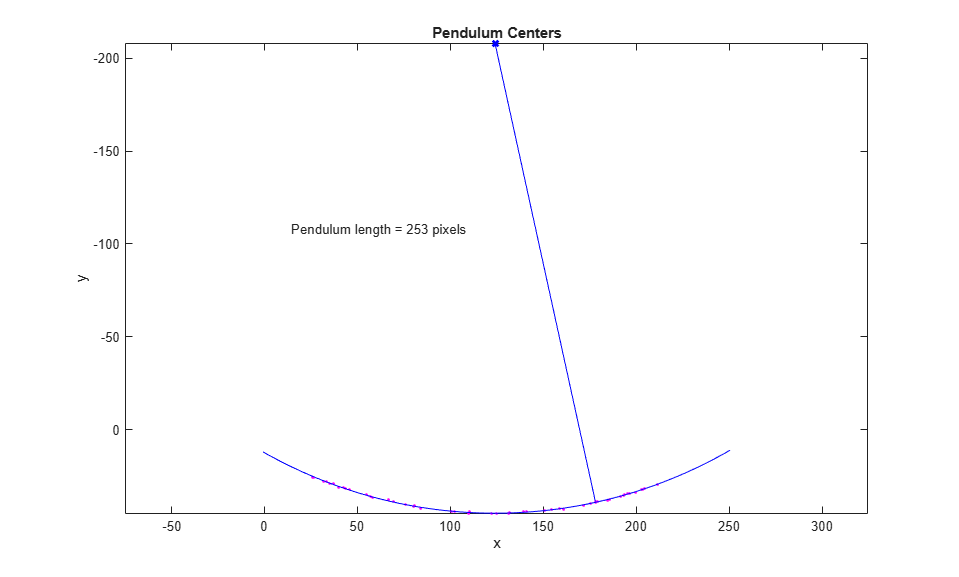## Finding the Length of a Pendulum in Motion

This example shows you how to calculate the length of a pendulum in motion. You can capture images in a time series with the Image Acquisition Toolbox™ and analyze them with the Image Processing Toolbox™.

### Step 1: Acquire Images

Load the image frames of a pendulum in motion. The frames in the MAT-file `pendulum.mat` were acquired using the following functions in the Image Acquisition Toolbox.

```% Access an image acquisition device (video object). % vidimage=videoinput('winvideo',1,'RGB24_352x288'); % Configure object to capture every fifth frame. % vidimage.FrameGrabInterval = 5; % Configure the number of frames to be logged. % nFrames=50; % vidimage.FramesPerTrigger = nFrames; % Access the device's video source. % src=getselectedsource(vidimage); % Configure device to provide thirty frames per second. % src.FrameRate = 30; % Open a live preview window. Focus camera onto a moving pendulum. % preview(vidimage); % Initiate the acquisition. % start(vidimage); % Wait for data logging to finish before retrieving the data. % wait(vidimage, 10); % Extract frames from memory. % frames = getdata(vidimage); % Clean up. Delete and clear associated variables. % delete(vidimage) % clear vidimage % load MAT-file load pendulum; ```

### Step 2: Explore Sequence with IMPLAY

Run the following command to explore the image sequence in `implay`.

```implay(frames); ```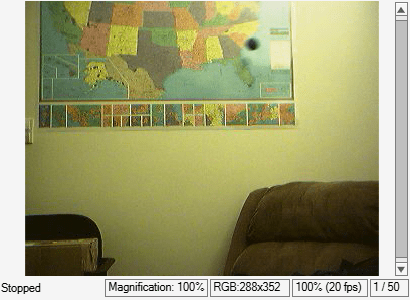### Step 3: Select Region where Pendulum is Swinging

You can see that the pendulum is swinging in the upper half of each frame in the image series. Create a new series of frames that contains only the region where the pendulum is swinging.

To crop a series of frames using `imcrop`, first perform `imcrop` on one frame and store its output image. Then use the previous output's size to create a series of frame regions. For convenience, use the `rect` that was loaded by `pendulum.mat` in `imcrop`.

```nFrames = size(frames,4); first_frame = frames(:,:,:,1); first_region = imcrop(first_frame,rect); frame_regions = repmat(uint8(0), [size(first_region) nFrames]); for count = 1:nFrames frame_regions(:,:,:,count) = imcrop(frames(:,:,:,count),rect); end imshow(frames(:,:,:,1)) ```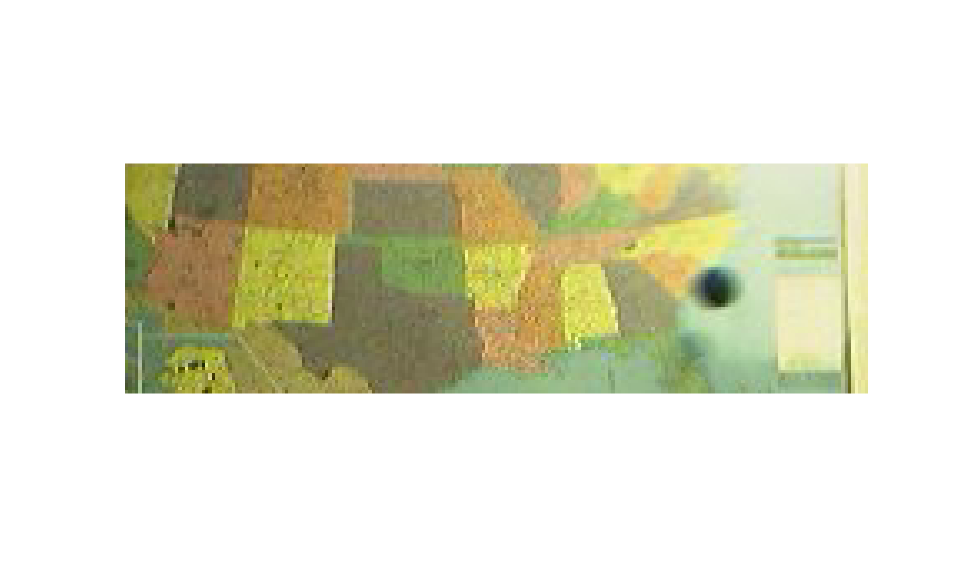### Step 4: Segment the Pendulum in Each Frame

Notice that the pendulum is much darker than the background. You can segment the pendulum in each frame by converting the frame to grayscale, thresholding it using `imbinarize`, and removing background structures using `imopen` and `imclearborder`.

initialize array to contain the segmented pendulum frames.

```seg_pend = false([size(first_region,1) size(first_region,2) nFrames]); centroids = zeros(nFrames,2); se_disk = strel('disk',3); for count = 1:nFrames fr = frame_regions(:,:,:,count); gfr = rgb2gray(fr); gfr = imcomplement(gfr); bw = imbinarize(gfr,.7); % threshold is determined experimentally bw = imopen(bw,se_disk); bw = imclearborder(bw); seg_pend(:,:,count) = bw; montage({fr,labeloverlay(gfr,bw)}); pause(0.2) end ```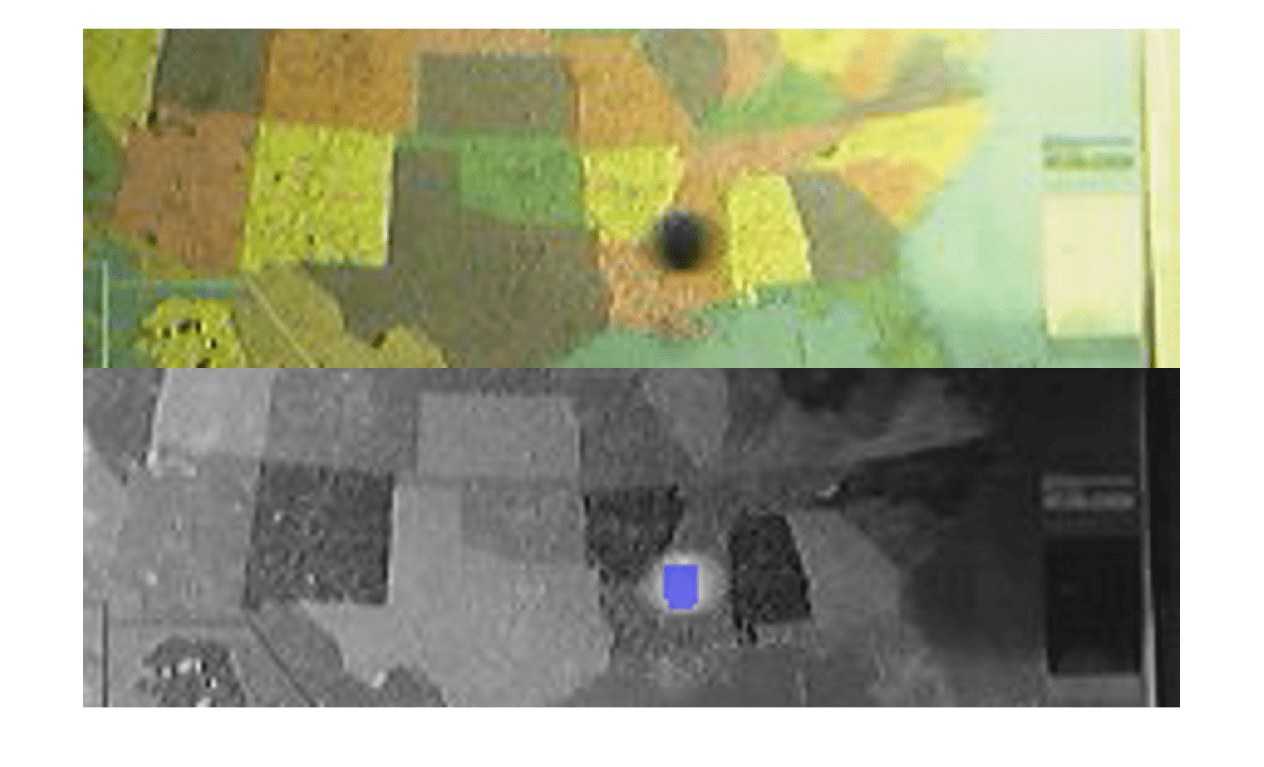### Step 5: Find the Center of the Segmented Pendulum in Each Frame

You can see that the shape of the pendulum varied in different frames. This is not a serious issue because you just need its center. You will use the pendulum centers to find the length of the pendulum.

Use `regionprops` to calculate the center of the pendulum.

```pend_centers = zeros(nFrames,2); for count = 1:nFrames property = regionprops(seg_pend(:,:,count), 'Centroid'); pend_centers(count,:) = property.Centroid; end ```

Display pendulum centers using `plot`.

```x = pend_centers(:,1); y = pend_centers(:,2); figure plot(x,y,'m.') axis ij axis equal hold on; xlabel('x'); ylabel('y'); title('Pendulum Centers'); ```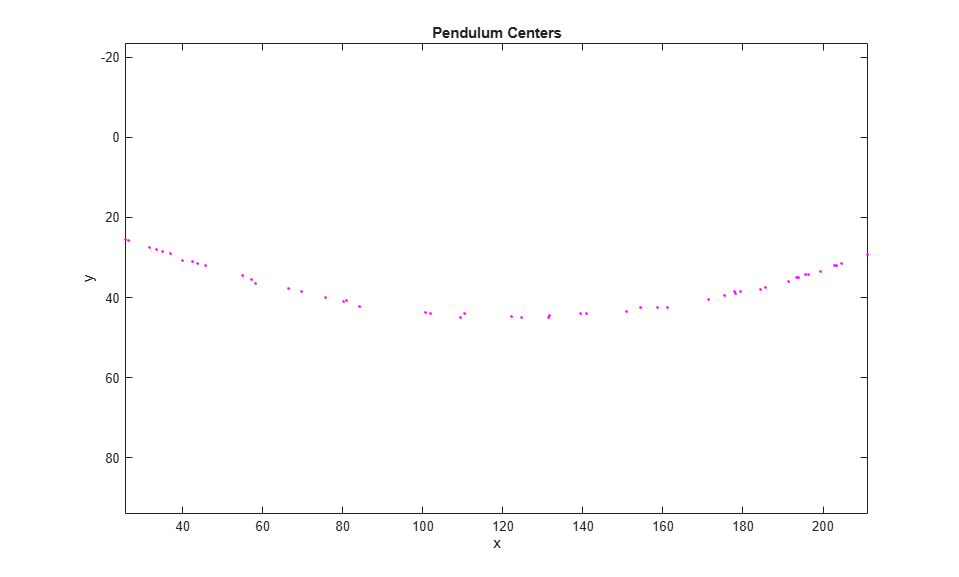### Step 6: Calculate Radius by Fitting a Circle Through Pendulum Centers

Rewrite the basic equation of a circle:

`(x-xc)^2 + (y-yc)^2 = radius^2`

where `(xc,yc)` is the center, in terms of parameters `a`, `b`, `c` as

`x^2 + y^2 + a*x + b*y + c = 0`

where `a = -2*xc`, `b = -2*yc`, and `c = xc^2 + yc^2 - radius^2`.

You can solve for parameters `a`, `b`, and `c` using the least squares method. Rewrite the above equation as

`a*x + b*y + c = -(x^2 + y^2)`

which can also be rewritten as

`[x y 1] * [a;b;c] = -x^2 - y^2`.

Solve this equation using the backslash(`\`) operator.

The circle radius is the length of the pendulum in pixels.

```abc = [x y ones(length(x),1)] \ -(x.^2 + y.^2); a = abc(1); b = abc(2); c = abc(3); xc = -a/2; yc = -b/2; circle_radius = sqrt((xc^2 + yc^2) - c); pendulum_length = round(circle_radius) ```
```pendulum_length = 253 ```

Superimpose circle and circle center on the plot of pendulum centers.

```circle_theta = pi/3:0.01:pi*2/3; x_fit = circle_radius*cos(circle_theta)+xc; y_fit = circle_radius*sin(circle_theta)+yc; plot(x_fit,y_fit,'b-'); plot(xc,yc,'bx','LineWidth',2); plot([xc x(1)],[yc y(1)],'b-'); text(xc-110,yc+100,sprintf('Pendulum length = %d pixels', pendulum_length)); ```